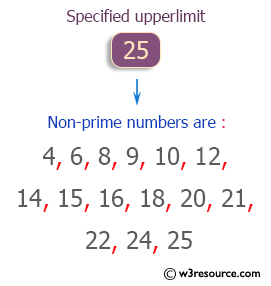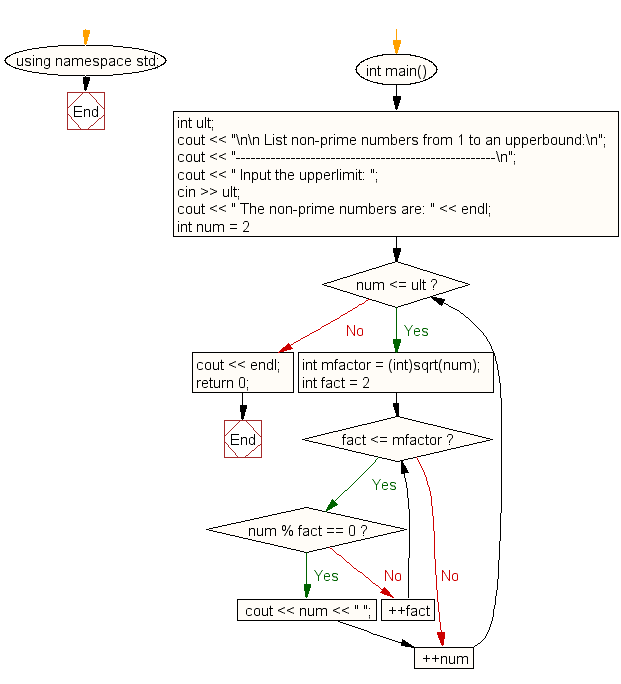﻿ C++ Exercises: List non-prime numbers from 1 to an upperbound - w3resource# C++ Exercises: List non-prime numbers from 1 to an upperbound

## C++ For Loop: Exercise-16 with Solution

Write a program in C++ to list non-prime numbers from 1 to an upperbound.

Pictorial Presentation:Sample Solution :-

C++ Code :

``````#include <iostream>
#include <cmath>
using namespace std;

int main()
{
int ult;
cout << "\n\n List non-prime numbers from 1 to an upperbound:\n";
cout << "----------------------------------------------------\n";
cout << " Input the upperlimit: ";
cin >> ult;
cout << " The non-prime numbers are: " << endl;
for (int num = 2; num <= ult; ++num)
{
int mfactor = (int)sqrt(num);
for (int fact = 2; fact <= mfactor; ++fact)
{
if (num % fact == 0)
{
cout << num << " ";
break;
}
}
}
cout << endl;
return 0;
}
``````

Sample Output:

``` List non-prime numbers from 1 to an upperbound:
----------------------------------------------------
Input the upperlimit: 25
The non-prime numbers are:
4 6 8 9 10 12 14 15 16 18 20 21 22 24 25
```

Flowchart:C++ Code Editor:

Contribute your code and comments through Disqus.

What is the difficulty level of this exercise?

﻿

## C++ Programming: Tips of the Day

What is a smart pointer and when should I use one?

This answer is rather old, and so describes what was 'good' at the time, which was smart pointers provided by the Boost library. Since C++11, the standard library has provided sufficient smart pointers types, and so you should favour the use of std::unique_ptr, std::shared_ptr and std::weak_ptr.

There was also std::auto_ptr. It was very much like a scoped pointer, except that it also had the "special" dangerous ability to be copied - which also unexpectedly transfers ownership.

It was deprecated in C++11 and removed in C++17, so you shouldn't use it.

```std::auto_ptr<MyObject> p1 (new MyObject());
std::auto_ptr<MyObject> p2 = p1; // Copy and transfer ownership.
// p1 gets set to empty!
p2->DoSomething(); // Works.
p1->DoSomething(); // Oh oh. Hopefully raises some NULL pointer exception.
```

Ref : https://bit.ly/3mc9GHE